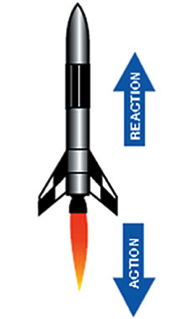Forces and Motion

Equation

Force = mass x acceleration

F = ma

1.18

Equation

weight = mass x acceleration of gravity

w = m x g

1.18

Newton's Laws of Motion

Isaac Newton, whose fingerprints are all over classical physics, developed three laws of motion that still fundamental.

Greek philosophers and other early scientists observed that whenever an object was moved – say a kicked ball – it stops ultimately. They assumed that all movement comes to an end. Newton had the insight to reach the opposite conclusion – all movement continues unless some force acts to stop it. This is formalized as his first law of motion:

Newton’s First Law of Motion:

An object at rest tends to stay at rest, and an object in motion tends to stay in motion in a straight line at a constant speed.

The word inertia is used to describe the tendency of an object to resist change in its motion. It’s a physics word, but also used in common talking – we all know someone who can’t overcome their inertia and continues to sit in front of a TV.

The amount of inertia a body possesses is measured by its mass. So a rolling bowling ball has a lot more inertia than a marble moving at the same speed.

Newton’s Second Law of Motion:

If you want to move a resting mass you have to apply a force, and the force needed to move a bowling ball is a lot more than what is needed to move a marble.

Newton expressed this as one of the most famous equations in physics:

Force = mass x acceleration   F = ma

Image: relative size of marbles and bowling ball fromhttp://blogtown.portlandmercury.comIf a bowling ball has a mass of 5 kg, and a marble’s mass is 5 g (=0.005 kg), to give each an acceleration of 2 m/s2 requires greatly difference forces:

For a bowling ball: F = ma = 5 kg x 2 m/s2 = 10 N

For a marble:  F = ma = 0.005 kg x 2 m/s2 = 0.01 N

Remember from the discussion of Eqn 1.10 that N stands for a Newton, which is the unit of force, being 1 kg*(m/s2).

10 N is 1000 times more force than 0.01 N, so you exert 1000 times more effort to sling a bowling ball down a lane than to use your thumb to propel a marble into a circle.

Students (and everyone else other than physics teachers) often confuse mass and weight. Mass is a measure of the inertia in a body, or essentially how many atoms are in it. The mass of an object doesn’t change unless more is added or some is taken away. Weight is a force due to the pull of gravity on a mass. As an equation this would be:

weight = mass x acceleration of gravity  w = m x g

This equation doesn’t get a number because it is exactly the same as Eqn 1.18. Remember weight is a force (F) and gravity is an acceleration (a), so yes, F = ma.

On Earth, the acceleration due to gravity is 9.8 m/s2 so the weight of something on Earth is 9.8 m/s2 multiplied by the mass. If your mass on Earth is 45 kg, your weight is 45 kg x 9.8 m/s2 = 445 N (100 lbs).

The quickest way to lose weight is not to exercise or diet, but to go to the Moon. On the Moon the acceleration of gravity is only 1.6 m/s2 so that your weight is

w = 45 kg x 1.6 m/s2 = 72 N (16 lbs)

Of course, if your rocket goes off course and you end up at Jupiter (g = 26 m/s2), you become a real pig:

w = 45 kg x 26 m/s2 = 1,170 N (263 lbs)

But no matter where you go, your mass remains 45 kg. Mass is constant, weight depends on gravity.

Mass and weight wouldn’t be confusing except that we use weight in everyday speech differently than we do in physics.  We say weight where a physicist would say mass.

Newton’s Third Law of Motion:You might have expected that if Newton had two laws he was such an overachiever that he would probably find a third one. The third law is so famous that we have all heard it:

For every action there is an equal and opposite reaction.

This is often illustrated with the example of a rocket. As the fuel inside it ignites, it exerts a force – an action - blowing the gases of the exhaust out of the rocket’s nozzle. The equal and opposite reaction is a force in the opposite direction that pushes the rocket forward.

One thing people are often confused over with the Third Law is that if you are skydiving, and gravity is accelerating you towards the Earth, what is the equal and opposite reaction? Your mass, puny as it is, is accelerating the Earth toward you. But because the Earth’s mass is vastly more than your mass, the acceleration you induce in the Earth is extraordinarily small, but it is still there.

Image: NASA graphic